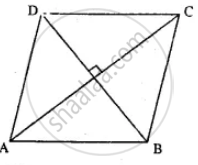# Abcd is a Parallelogram. What Kind of Quadrilateral is It If: Ac is Perpendicular to Bd but is Not Equal to It? - Mathematics

Sum

ABCD is a parallelogram. What kind of quadrilateral is it if: AC is perpendicular to BD but is not equal to it?

#### SolutionAC ⊥ BD (Given)

But AC & BD are not equal

∴ ABCD is a Rhombus.

Concept: Types of Quadrilaterals - Properties of a Parallelogram
Is there an error in this question or solution?

#### APPEARS IN

Selina Concise Mathematics Class 8 ICSE
Chapter 17 Special Types of Quadrilaterals
Exercise 17 | Q 11.2 | Page 198